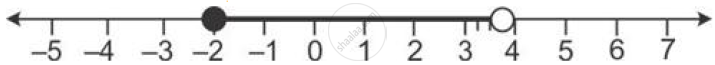# Solve the Following in Equation and Represent the Solution Set on the Number Line. R - 3 < -1/2 - (2x)/3 <= 5/6, X ∈ R - Mathematics

Solve the following in equation and represent the solution set on the number line.

R - 3 < -1/2 - (2x)/3 <= 5/6, x ∈ R

#### Solution

-3 < -1/2 - (2x)/3 <= 5/6

Multiply by 6, we get

=> -18 < -3-4x <= 5

=> -15 < -4x <= 8

Dividing by -4 we get

=> (-15)/(-4) > x >= 8/(-4)

=> -2 <= x < 15/4

=> x ∈ [-2, 15/4]Concept: Representation of Solution on the Number Line
Is there an error in this question or solution?